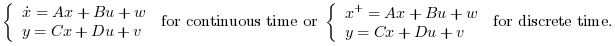Scilab Home page | Wiki | Bug tracker | Forge | Mailing list archives | ATOMS | File exchange
Scilab 6.1.0
Change language to: English - Français - 日本語 - Русский
Ajuda do Scilab >> CACSD > Control Design > Linear Quadratic > lqg2stan

# lqg2stan

LQG to standard problem

### Syntax

[P_aug, r] = lqg2stan(P, Qxu, Qwv)

### Arguments

P

State space representation of the nominal plant (nu inputs, ny outputs, nx states).

Qxu

[Q, S ; S', N] symmetric nx+nu by nx+nu weighting matrix.

Qwv

[R,T;T',V] symmetric nx+ny by nx+ny covariance matrix.

r

Row vector [ny nu].

P_aug

Augmented plant state space representation (see: syslin)

### Description

lqg2stan returns the augmented plant for linear LQG (H2) controller design problem defined by:

• The nominal plant P: described by• The (instantaneous) cost function.

• The noises covariance matrix### Algorithm

If [B1; D21] is a factor of Qxu, [C1, D12] is a factor of Qwv (see: fullrf) then

P_aug = syslin(P.dt, P.A, [B1,P.B], [C1;-P.C], [0,D12;D21,P.D])

### Examples

ny = 2;
nu = 3;
nx = 4;
P = ssrand(ny,nu,nx);
Qxu = rand(nx+nu,nx+nu);
Qxu = Qxu * Qxu';
Qwv = rand(nx+ny,nx+ny);
Qwv = Qwv * Qwv';
[P_aug, r] = lqg2stan(P, Qxu, Qwv);
K = lqg(P_aug,r);          // K=LQG-controller
spec(h_cl(P_aug, r, K))    // Closed loop should be stable
//Same as Cl = P/.K; spec(Cl('A'))

lqg2stan(1/(%s+2), eye(2,2), eye(2,2))

• lqg — LQG compensator
• lqr — LQ compensator (full state)
• lqe — linear quadratic estimator (Kalman Filter)
• obscont — observer based controller
• h_inf — Continuous time H-infinity (central) controller
• augment — augmented plant
• fstabst — Youla's parametrization of continuous time linear dynamical systems
• feedback — feedback operation

### History

 Version Description 6.0 It is no longer necessary to enter -P to get P_aug instead of -P_aug (bug 13751 fixed).